cancel

### Note on archived topics.

This topic has been archived. Information and links in this thread may no longer be available or relevant.
If you have a question create a new topic by clicking here and select the appropriate board.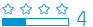Intern
53 40 0 4
Message 1 of 7
4,891
Flag PostHP Recommended

Solved!

# Issues writing equations with existing variables in Hp 50g

Hello,

I'm studying for June's exams and I'm trying to write the long equations in my HP (Algebraic, std, degress, rectangular), but whenever I try to write a new one, if there is a variable with the same name, it displays its value and no the name I typed. I know it might sound confusing, but here is an example:

• I'm doing an exercise and I save the next equation: A=B+C (as an example).
• I use it and I begin with a new exercise.
• In this exercise I save the equation: B=C*E*R/T, but when I try to save it into a variable it changes the letters B & C for theirs values. So if I want to solve an equation with different B & C values I can't because they don't appear on the "Solve Equation".

I know I can purge all the variables before writing a new equation but it is a pain in the ass, is there a proper way to do it?

Thanks.

Tags (10)
6 REPLIES 6
Highlighted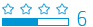414 341 11 136
Message 2 of 7
4,869
Flag PostHP Recommended

Solved!

## Issues writing equations with existing variables in Hp 50g

Hi!, JamesJames:

In your first Equation, you can store it, in the HOME (VAR), as global variable with any name ... 'EQ' and press, STO.

You must see, in the SOLVE EQUATION ... with random any values for B and C and result of ANow, you needed create other equation, with the values, B and C ... 'B=C*E*R/T'

You can store it with the equal name of previous ('EQ' and STO), or with other name.

If select equal name, you must see ...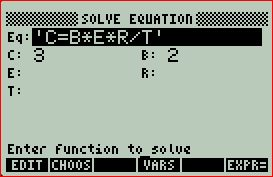Now, if you needed obtein the value of C ...

Complete the other values, moving plated arrows down, right or left, according to requirement.

p.e. : E= 2.5; R= 6 and T= 0.5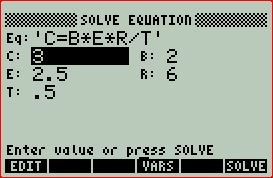Now, you needed go with the up plated arrow to C and press F6 (SOLVE). Then you must see, the result of C.Note: If you needed erase any global variable, you can use ...

1: {the variable} PURGE

Example: If you decide PURGE all global variables ...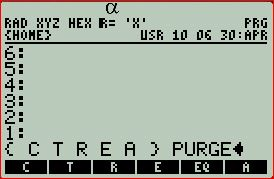or ...Only needed press F5 (PURGE).

See, the Learnin modules Using the Equation Writer, from ... http://h20331.www2.hp.com/Hpsub/cache/383680-0-0-225-121.html178 177 17 80
Message 3 of 7
4,858
Flag PostHP Recommended

Solved!

## Issues writing equations with existing variables in Hp 50g

@Jamesjames wrote:

I'm studying for June's exams and I'm trying to write the long equations in my HP (Algebraic, std, degress, rectangular), but whenever I try to write a new one, if there is a variable with the same name, it displays its value and no the name I typed. I know it might sound confusing, but here is an example:

• I'm doing an exercise and I save the next equation: A=B+C (as an example).
• I use it and I begin with a new exercise.
• In this exercise I save the equation: B=C*E*R/T, but when I try to save it into a variable it changes the letters B & C for theirs values. So if I want to solve an equation with different B & C values I can't because they don't appear on the "Solve Equation".

You will not have to use purge with this method.

your first equation would be entered as:  (use either equation writer or the command line)

'A=B+C'

STO>'EQ1'

(for this example, when "solve equation" was executed for the equation A=B+C, a value of 8 was entered for 'B' and a value of 9 was entered for 'C' )

NOW THE IMPORTANT STEP....  (this step is not required when operating in RPN mode ... In RPN mode, just enter the equation using EQW or the commmand line as normally done)

when entering the second equation,  this can not be easily done using Equation Writer.

To easily solve the problem of entering the second equation and keeping the variables un-altered in the equation you must enter the equations on the command line.

start with a single quotation.... this tells the 50G that an equation will be entered

when entering the variables that have been previously used... put single quotations around those variables...

for example:

'  'B' = 'C' * E*R/T '

press ENTER

your screen should look like this::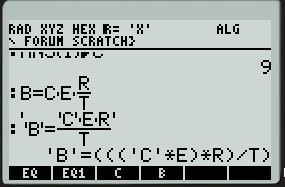now

STO> 'EQ'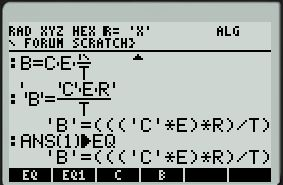Enter "Solve Equation" and the variables used before are displayed with their values pre-populated... but all variable can be changed...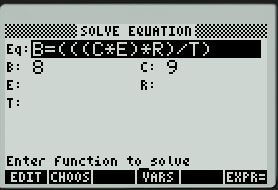NOTE NOTE:: if you want to change equations in this window, just use the CHOOS key, select the other equation.... in this example it is called EQ1...(The CHOOS softkey selection is only available when the Equation is highlighted as shown in the above screen)

press CHOOS and see the following ::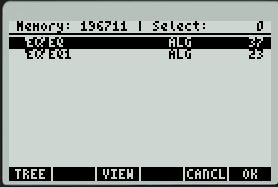highlight EQ1 and press F6 (OK)

now the screen changes::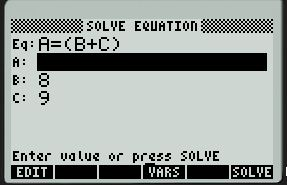414 341 11 136
Message 4 of 7
4,841
Flag PostHP Recommended

Solved!

## Issues writing equations with existing variables in Hp 50g

Hi!, JamesJames:

This is, other metod, for SOLVE ...

Configure FLAG ...

03 Function -> num

Both equation's, are stored with 'EQ' name, but the program, you must stored with other name, p.e.: 'PRB'.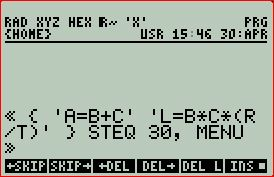Put each value of 'B' and 'C' and press F2 and F3; in F4 (EXPR=), you can obtein the result ...

Now, press F6 (NXEQ) and you can see, the other equation ...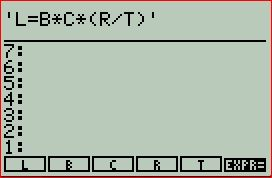Only needed put the values in only, R and T and press ... F6 (EXPR=)Intern
53 40 0 4
Message 5 of 7
4,752
Flag PostHP Recommended

Solved!

## Issues writing equations with existing variables in Hp 50g

Thank you both, both answers have been very helpful. I don't know if I should start a new thread but... here I go: I'm trying to writein the Equation Writer as a variable but I can't, it appears as.178 177 17 80
Message 6 of 7
4,728
Flag PostHP Recommended

Solved!

## Issues writing equations with existing variables in Hp 50g

@Jamesjames wrote:

I don't know if I should start a new thread but... here I go: I'm trying to writein the Equation Writer as a variable but I can't, it appears as.

yes, for the benefit of all future forum users, next time, please start a new thread.   It makes it easier for future users to search by topic.

The referenced variable you are describing is not entered into the 50G as a "subscripted" variable.

These variable types are entered as an array variable.

This is the correct process for Algebraic or RPN mode:

So in equation writer, enter the variable in question as follows:

RIGHT SHIFT

EQW (the single quotation key)

ALPHA

G

LEFT SHIFT

@( )   @the "subtraction" key

ALPHA

i

+

1

ENTER

STO>EQ22

NOTE that the variable "G" is an considered by the 50G as an array of numbers.

NOTE NOTE:: for Algebraic users only,  if the variable "i" has already been created and stored, the variable G(i+1) must be created on the command line using single quotations as was shown earlier in this post.

Using MTRW  (the matrix writer) you can create the vector (array) as follows

LEFT SHIFT

MTRW  (the single quotation key)

enter some numbers as shown::STO>'G'

now store a value for variable for "i"  ... for this example, the number 2

now EVAL EQ22 ...

since i=2, this should return G(3) = 3

EVAL

softkey for EQ22

ENTER

result:   3178 177 17 80
Message 7 of 7
4,655
Flag PostHP Recommended

Solved!

## Issues writing equations with existing variables in Hp 50g

@pin224466 wrote:

To easily solve the problem of entering the second equation and keeping the variables un-altered in the equation you must enter the equations on the command line.

The equation writer can be used in Algebraic mode to enter formulas and keep the variable un-altered.  However, it requires an extra step when using it.

This method will allow easy entry of more complicated functions when variables are used in multiple equations....

using your original example, one equation is entered as 'A=B+C'  and "solve equation" has been execute.

now enter a second equation this time using Equation Writer

RIGHT SHIFT
EQW

now enter the equation B=C*sin(E)*R /sqrt T     as shown: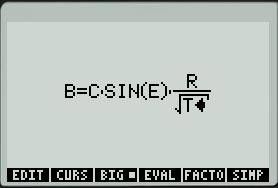now press ENTER   only 1 time !

The display should look like the following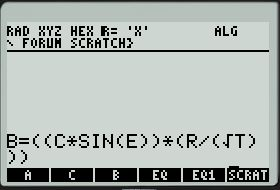now use the LEFT ARROW key to navigate the cursor to the left side of the variable "B"

Press the single quotation key.

since this will automatically enter qty 2 single quotations, on of the single quotation must be deleted using the backspace key

now use the RIGHT ARROW key to navigate the cursor to the right side of the variable "B"

Press the single quotation key.

since this will automatically enter qty 2 single quotations, on of the single quotation must be deleted using the backspace key

repeat the process and put single quotations around the variable "C"

your screen should look like the following: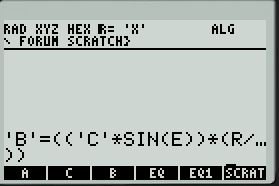now press ENTER

then execute

STO>'EQ'

and save the equation.

execute "solve equation" as in the previous post...

### Note on archived topics.

This topic has been archived. Information and links in this thread may no longer be available or relevant.
If you have a question create a new topic by clicking here and select the appropriate board.
† The opinions expressed above are the personal opinions of the authors, not of HP. By using this site, you accept the Terms of Use and Rules of Participation